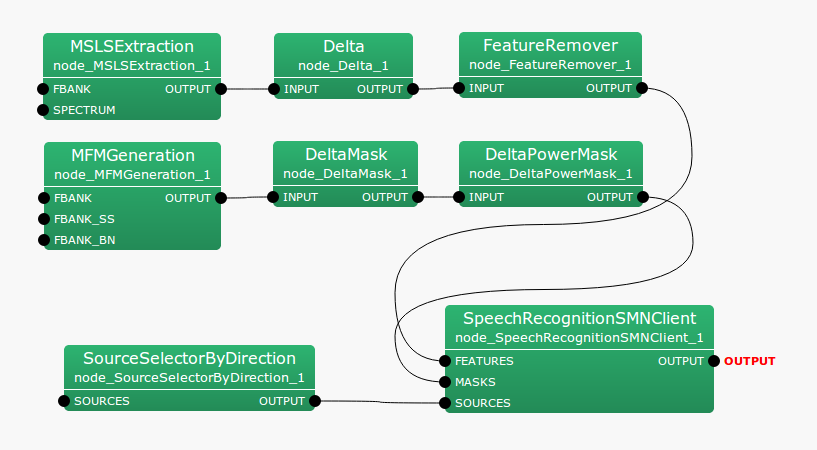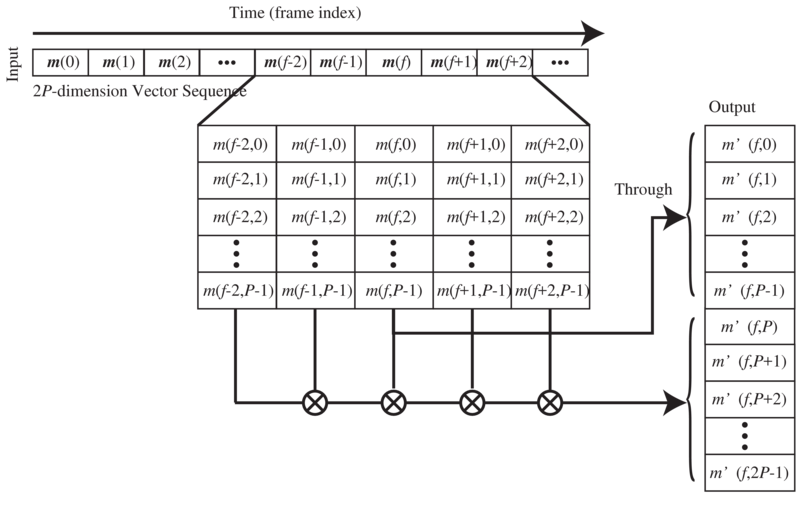### 6.5.1.1 Outline of the node

This node obtains dynamic missing feature mask vectors from static missing feature mask vectors. It generates mask vectors consisting of the missing feature mask vectors of static and dynamic features.

### 6.5.1.2 Necessary file

No files are required.

### 6.5.1.3 Usage

When to use

This node is used to perform speech recognition by masking features depending on reliability based on the missing feature theory. It is usually used for the latter half of MFMGeneration .

Typical connectionFigure 6.82: Typical connection example of DeltaMask

### 6.5.1.4 Input-output and property of the node

Table 6.70: Parameter list of DeltaMask
 Parameter name Type Default value Unit Description FBANK_COUNT Dimension number of static feature

Input

INPUT

: Map<int, ObjectRef> type. A sound source ID and feature mask vector (of Vector<float> type) data pair. The mask value is a real number from 0.0 to 1.0. 0.0 indicates the feature is not reliable and 1.0 indicates it is reliable.

Output

OUTPUT

: Map<int, ObjectRef> type. A pair of the sound source ID and mask vectorof the feature as Vector<float> type data. The mask value is a real number from 0.0 to 1.0. 0.0 indicates the feature is not reliable and 1.0 indicates it is reliable.

Parameter

FBANK_COUNT

: int  type. The number of feature dimensions to process. Its range is positive integer.

### 6.5.1.5 Details of the node

This node obtains missing feature mask vectors of dynamic features from those of static features and generates mask vectors consisting of the missing feature mask vectors of static and dynamic features. The input mask vector at the frame time $f$ is expressed as;

 $\displaystyle \boldsymbol {m}(f)$ $\displaystyle =$ $\displaystyle [m(f,0),m(f,1),\dots ,m(f,2P-1)] ^{T}$ (130)

Here, $P$ indicates the number of dimensions of static features among the input mask vectors and is given in FBANK_COUNT. The mask values for the dynamic features are obtained from those of the static features: the output is substituted into the dimensional elements from $P$ to $2P-1$. The output vector $\boldsymbol {m}’(f)$ is expressed as follows.

 $\displaystyle \boldsymbol {y}’(f)$ $\displaystyle =$ $\displaystyle [m’(f,0),m’(f,1),\dots ,m’(f,2P-1)]^{T}$ (131) $\displaystyle m’(f,p)$ $\displaystyle =$ $\displaystyle \left\{ \begin{array}{ll}m(f,p), & {if~ ~ } p=0, \dots , P-1, \\ \displaystyle \prod _{\tau =-2}^{2} m(f+\tau ,p), & {if~ ~ } p=P, \dots , 2P-1, \end{array} \right.$ (132)

Figure 6.83 shows an input-output flow of DeltaMask .Figure 6.83: Input-output flow of DeltaMask .